# Q1 - Two identical blocks are attached to identical springs. The oscillation amplitude of Block A...

Q1 - Two identical blocks are attached to identical springs. The oscillation amplitude of Block A is 10cm; and the amplitude of Block B is 5 cm. Which block has a greater oscillation period?

 A- Block A B- Block B C- The periods are the same for both Blocks.

Q2 - For an object in oscillation, which of the following is defined to be the maximum displacement from the equilibrium position.

 A- Period B- Frequency C- Angular frequency D- Amplitude

Q3 - A simple pendulum's period is 6.51 seconds . What is the angular frequency in rad/s?

Q4 - An object oscillates with an angular frequency of 2.02 /s and an amplitude 0.13 m. What is the magnitude of its maximun acceleration in?

Q5 - Which of the sensors must be zeroed before making measurement in this experiment?

 A. Just motion sensor. B. Just force sensor C. Both motion and force sensor D. Motion sensor, force sensor and meter stick

Q1. C

given two identical blocks are attached to identical springs

and amplitude of Block A is 10cm; and the amplitude of Block B is 5 cm

we know that the time period is T = 2pi(sqrt(m/k))

so in the expression of Time period there is no amplitude term so the time period does not depend on amplitude.

answer is C The periods are the same for both Blocks.

Q2 D

For an object in oscillation,the maximum displacement from the equilibrium position defined to be the Amplitude

Q3

T = 6.51 s

the angular frequency is w = 2pi/T = 2pi/6.51 raad/s = 0.965159 rad/s

Q4

angular frequency W = 2.02 /s , amplitude A = 0.13 m

the magnitude of its maximun acceleration is a = ?

we know that F = -k*x

for maximum amplitude F = -kA = m*a

= - m*w^2*A = ma ==> a = W^2*A

the maximum acceleration is a = 2.02^2*0.13 m/s2 = 0.530452 m/s2

Q5

force sensor should be zeroed

#### Earn Coin

Coins can be redeemed for fabulous gifts.

Similar Homework Help Questions
• ### bead block 4) Bead on a Block A block is attached to a spring so that...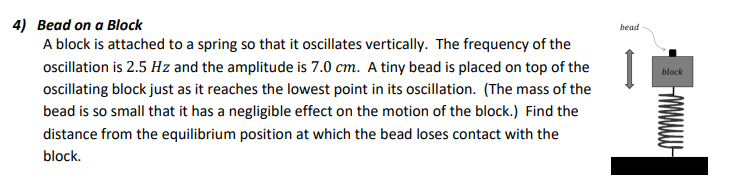bead block 4) Bead on a Block A block is attached to a spring so that it oscillates vertically. The frequency of the oscillation is 2.5 Hz and the amplitude is 7.0 cm. A tiny bead is placed on top of the oscillating block just as it reaches the lowest point in its oscillation. (The mass of the bead is so small that it has a negligible effect on the motion of the block.) Find the distance from the equilibrium...

• ### 1) The drawing shows three identical springs hanging from the ceiling. Nothing is attached to the...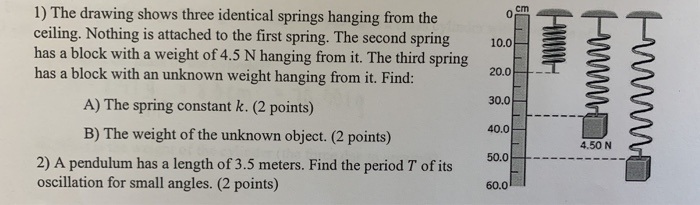1) The drawing shows three identical springs hanging from the ceiling. Nothing is attached to the first spring. The second spring has a block with a weight of 4.5 N hanging from it. The third spring has a block with an unknown weight hanging from it. Find: A) The spring constant k. (2 points) B) The weight of the unknown object. (2 points) 2) A pendulum has a length of 3.5 meters. Find the period T of its oscillation for...

• ### please answer as many questions as possible. I will “thumb up” the answers. Thanks! 1. You are on a boat, which is bobbing up and down. The boat's vertical displacement y is given by y 1.2...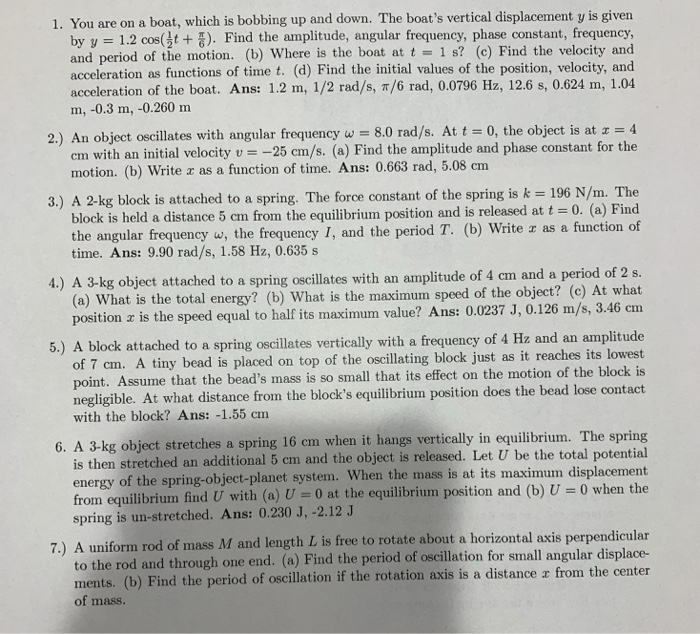please answer as many questions as possible. I will “thumb up” the answers. Thanks! 1. You are on a boat, which is bobbing up and down. The boat's vertical displacement y is given by y 1.2 cos(t). Find the amplitude, angular frequency, phase constant, frequency, and period of the motion. (b) Where is the boat at t 1 s? (c) Find the velocity and acceleration as functions of time t. (d) Find the initial values of the position, velocity, and...

• ### A 2.8 ?? block attached to a massless spring and is displaced 35 ?? by a...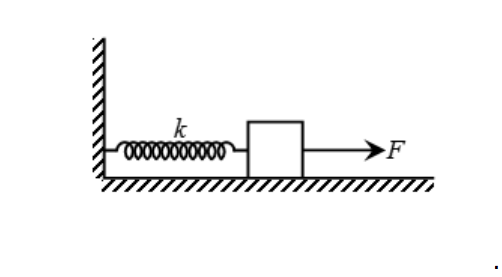A 2.8 ?? block attached to a massless spring and is displaced 35 ?? by a force of 927.5 ? across a smooth floor. The box is then let go and undergoes simple harmonic motion. Determine, a. The maximum acceleration of the block. b. The period of oscillation. c. The angular frequency. d. The speed at equilibrium. e. The velocity at ? = 16.2 ?�

• ### 10:06 MW W 1) The drawing shows three identical springs hanging from the ceiling. Nothing is...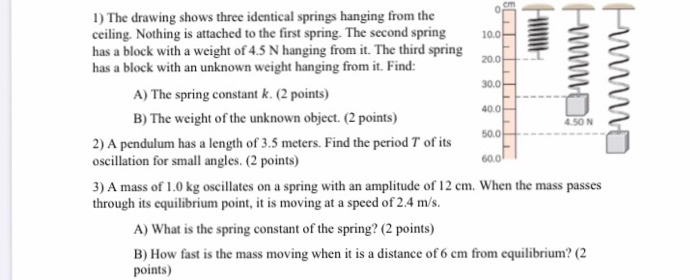10:06 MW W 1) The drawing shows three identical springs hanging from the ceiling. Nothing is attached to the first spring. The second spring has a block with a weight of 4.5 N hanging from it. The third spring has a block with an unknown weight hanging from it. Find: A) The spring constant k. (2 points) 40.04 B) The weight of the unknown object. (2 points) 500 - 2) A pendulum has a length of 3.5 meters. Find the...

• ### An oscillator consists of a block of mass 420 g connected to a spring. When set...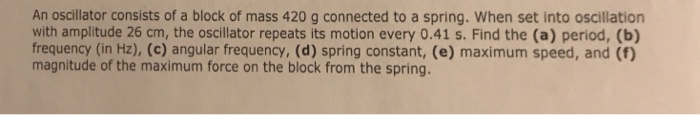An oscillator consists of a block of mass 420 g connected to a spring. When set into oscillation with amplitude 26 cm, the oscillator repeats its motion every 0.41 s. Find the (a) period, (b) frequency (in Hz), (c) angular frequency, (d) spring constant, (e) maximum speed, and (f) magnitude of the maximum force on the block from the spring.

• ### oscillation transfer device

You are to build the oscillation transfer device shown in Fig. 15-25. It consists of two spring–block systems hanging from a flexible rod. When the spring of system 1 is stretched and then released, the resulting SHM of system 1 at frequency f1 oscillates the rod. The rod then exerts a driving force on system 2, at the same frequency f1. You can choose from four springs with spring constants k of 1600, 1500, 1400, and 1200 N/m, and four...

• ### Chic Taupe Puffer Jacket DORI JS Review Submission History... WileyPLUS A Blocks Attached to AH + WileyPLUS: MyWile...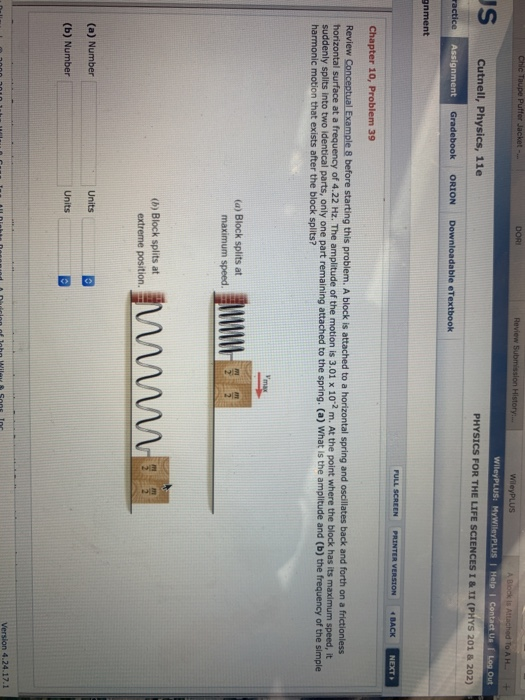Chic Taupe Puffer Jacket DORI JS Review Submission History... WileyPLUS A Blocks Attached to AH + WileyPLUS: MyWileyPLUS | Help Contact Us Log Out PHYSICS FOR THE LIFE SCIENCES I & II (PHYS 201 8 202) Cutnell, Physics, 11e ractice Assignment Gradebook ORION Downloadable eTextbook gnment FULL SCREEN PRINTER VERSION BACK NEXT Chapter 10, Problem 39 Review Conceptual Example 8 before starting this problem. A block is attached to a horizontal spring and oscillates back and forth on a frictionless...

• ### A mass of 500 grams is attached to two springs whose spring constants are k1=2 N/m...

A mass of 500 grams is attached to two springs whose spring constants are k1=2 N/m and k2 = 5 N/m, which are in turn attached to a wall. The system is on a horizontal frictionless surface. The system is displaced to the right and released. (a) What is the effective spring constant of the two springs in ”series”? Hint use Hooke’s law and the fact that the force required to displace the system is the same acting on each...

• ### Problems 36 and 90 m Block 1 46. Pre effective force constant of IJU torcycle, does...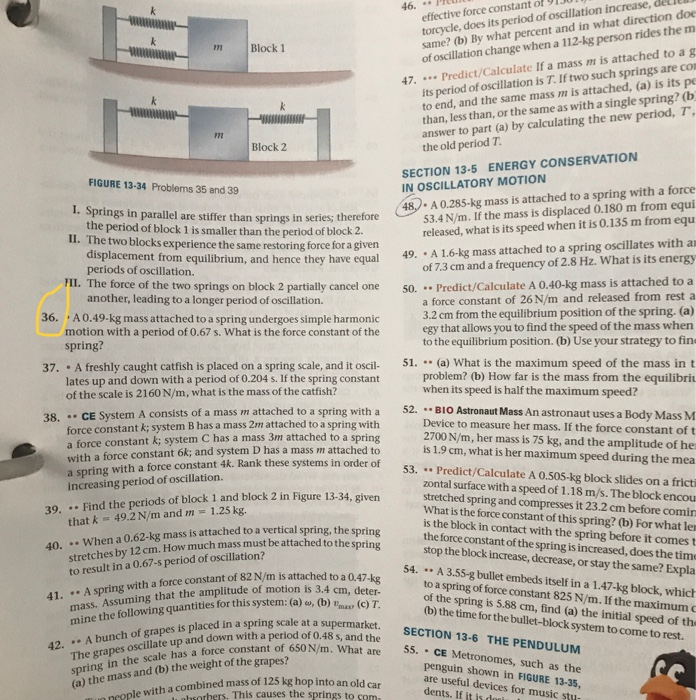Problems 36 and 90 m Block 1 46. Pre effective force constant of IJU torcycle, does its period of oscillation increase, delle same? (b) By what percent and in what direction doe of oscillation change when a 112-kg person rides them 47. .. Predict/Calculate If a mass m is attached to a g its period of oscillation is T. If two such springs are con to end, and the same mass m is attached, (a) is its pe than, less...